# SAS/ETS Examples

## Estimating a Consumption-Based Asset Pricing Model

Contents | SAS Program

# Overview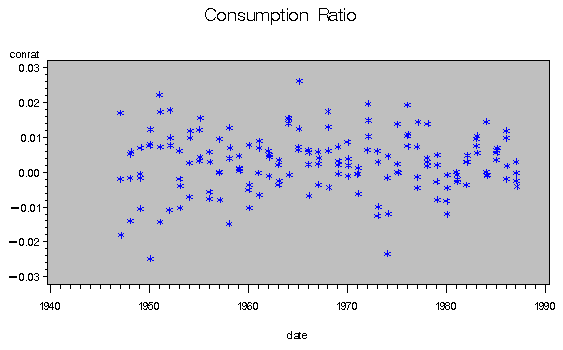The process by which assets are priced is one of the fundamental problems in financial economics. One approach, developed by Lucus (1978), asserts that individuals hold assets in order to optimize their intertemporal consumption. In his classic model, individuals seek to maximize a time-additive intertemporal discounted utility function that depends upon stochastic consumption.
If an asset has a real rate of return Rt, an individual may be able to increase his utility by deferring consumption from the current period and investing in the asset in order to consume in a later period. The relative attractiveness between current and future consumption affects the asset's price as reflected in its return. An important implication is that changes in consumption should mirror changes in asset prices.

Following Hansen and Singleton (1982), the agent maximizes the above utility function subject to the sequence of budget constraints
where N is the number of assets in the economy, Pit is the value of asset i in period t (price plus any cash payout), Qit is the amount of asset i owned by the individual at the end of period t, and Wt is real labor income at date t.

The first-order conditions yield the following Euler equations
where Rt+1 is 1 plus the real rate of return on asset i, (Pi,t+1/Pi,t), and U' is marginal utility. This implies that, in equilibrium, the value of an asset is its discounted future payoff weighted by the trade-off between future and present consumption, conditioned on the public information set at time t, It.

By assuming a specific utility function you can relate the Euler equation that characterizes the agent's optimal solution to the returns that you see in the market.

Assuming a power utility function [Note: this is a restrictive assumption. Refer to Pratt (1964) for a discussion on risk-aversion and utility functions.] with risk aversion coefficient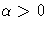, the above equation can be written in the estimable form
where Zt is a vector of instruments that represent the public's information set at time t. This yields a set of moment conditions corresponding to the equilibrium asset prices and provides the proper environment to apply Hansen's (1982) generalized method of moments (GMM) to estimate the risk-aversion and time-preference parameters.

# Analysis

With the assumption of a power utility function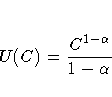you can estimate a simple consumption-based asset pricing model from the Euler equationsThe left side of this expression can be thought of as an error term ut+1 that should have conditional mean 0, given the information set at time t, under the hypothesis of rational expectations. This defines a set of orthogonality conditions E(ut+1 | Zt) = 0, implying E(ut+1Zt) = 0. If Zt is any subset of the variables in the current information set, these orthogonality conditions can be exploited by using Hansen's (1982) generalized method of moments (GMM) to estimate the parameters of the model and to test their implications.

Briefly, the GMM estimator is computed by minimizing the quadratic form

q = m' W-1 m
where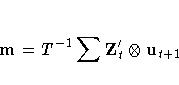and W is the asymptotic variance/covariance matrix for the orthogonality conditions m.

To perform the estimation requires data on some measure of consumption, returns for the assets of interest, and instruments from the public's information set. Of these, measures of consumption are the most difficult to obtain. Ferson and Harvey (1992) provide an informative discussion on the intricacies of consumption data.

The data for this example are the same as those used by Ferson and Harvey (1992) in estimating equation (3) from their paper. They use quarterly observations from the second quarter of 1947 (1947.6) through the fourth quarter of 1987 (1987.12). Seasonally adjusted nondurables deflated by seasonally adjusted personal consumption deflators are used as the measure of consumption; the four assets used are the real returns from government and corporate bonds and the smallest and largest equity decile real returns. Real returns are nominal returns deflated by the price index corresponding to the consumption growth measure. In addition, four lags of the real Treasury bill return and four lags of real consumption growth, both deflated by the overall Con sumer Price Index (CPI), are used as the instruments, Zt.

In the MODEL procedure, the endogenous variable is the percentage change in consumption, given by the variable CONRAT. The exogenous variables are the real returns for government bonds (GB), corporate bonds (CB), and the smallest and largest equity deciles (D1 and D10, respectively). One difference between the MODEL procedure and many other estimation tools is that the parameters to be estimated need to be specified explicitly. For this example, BETA denotes the time discount factor and ALPHA denotes the concavity parameter. Because computing parameter estimates for nonlinear equations requires an iterative process, you can supply the starting values. This example uses starting values of 1.0 for both parameters; the default starting values are 0.0001 for all parameters.

   proc model data=harvey;
endogenous conrat;
exogenous gb cb d1 d10 ;
parms beta 1.0 alpha 1.0;


You can also transform variables for use in the estimation. The following statements create the four lagged values of real consumption growth (CINST) and the real Treasury bill return (RINST) to be used as instruments in the model.

      lc1 = lag(cinst);
lc2 = lag2(cinst);
lc3 = lag3(cinst);
lc4 = lag4(cinst);
ltb1 = lag(rinst);
ltb2 = lag2(rinst);
ltb3 = lag3(rinst);
ltb4 = lag4(rinst);


The requisite moment conditions for the four assets are given by

      eq.h1 = beta * (1+conrat)**(-alpha) * (1+gb) - 1 ;
eq.h2 = beta * (1+conrat)**(-alpha) * (1+cb) - 1 ;
eq.h3 = beta * (1+conrat)**(-alpha) * (1+d1) - 1 ;
eq.h4 = beta * (1+conrat)**(-alpha) * (1+d10) - 1 ;


You fit these equations by specifying the iterated GMM option using a Parzen kernel. Iterated GMM re-estimates the variance matrix at each iteration with the parameters determined by the GMM estimation from the previous iteration. See Ferson and Foerster (1994) for a discussion of iterated GMM. Iteration terminates when the variance matrix for the equation errors changes by less than the value of the CONVERGE= option. The default convergence value is 0.001. The KERNEL= option enables you to specify the smoothing function for the variance matrix. The INSTRUMENTS statement specifies the instrumental variables to be used in the ITGMM estimation method. This example uses the lagged variables created above.

      fit h1-h4 / itgmm kernel=(parzen,1,0);
instruments lc1-lc4 ltb1-ltb4 ;
run;


 Consumption-Based Asset Pricing Model

 The MODEL Procedure

 Nonlinear ITGMM Summary of Residual Errors Equation DF Model DF Error SSE MSE Root MSE R-Square Adj R-Sq h1 0.5 158.5 0.4277 0.00270 0.0519 h2 0.5 158.5 0.4111 0.00259 0.0509 h3 0.5 158.5 3.1048 0.0196 0.1400 h4 0.5 158.5 0.9861 0.00622 0.0789

 Nonlinear ITGMM Parameter Estimates Parameter Estimate Approx Std Err t Value Approx Pr > |t| beta 1.001594 0.00390 256.80 <.0001 alpha 0.645325 0.8546 0.76 0.4513

 Number of Observations Statistics for System Used 159 Objective 0.2418 Missing 0 Objective*N 38.4454

For this simple model, the estimate of the time discount factor, BETA, is 1.00159 with an approximate standard error of 0.00390, while the estimate of the concavity parameter, ALPHA, is 0.64533 with an approximate standard error of 0.85462. In a time-separable model, such as this one, ALPHA can be thought of as the coefficient of relative risk aversion or as the inverse of the elasticity of consumption with respect to a fixed interest rate. The estimate of ALPHA in this example is not unusually large. These estimates are the same values reported by Ferson and Harvey (1992) in Panel B of Table IV in their paper, a four asset system with seasonally adjusted nondurables as the consumption measure. Any small differences can be attributed to the use of different kernel/bandwidth combinations.

Let r be the number of unique instruments times the number of equations. The value r represents the number of orthogonality conditions imposed by the GMM method. Under the assumptions of the GMM method, r - p linearly independent combinations of the orthogonality conditions should be close to 0. The GMM estimates are computed by setting these combinations to 0. When r exceeds the number of parameters to be estimated, the OBJECTIVE*N, reported at the end of the estimation, is an asymptotically valid statistic to test the null hypothesis that the over-identifying restrictions of the model are valid. The OBJECTIVE*N is distributed as a chi-square with r - p degrees of freedom. The p-value of the test can be calculated with the following DATA step.

   data _null_;
/* OBJECTIVE*N, degrees of freedom */
p = 1-probchi( 38.4454, 34 );
put p=;
run;


In this example, OBJECTIVE*N = 38.445 is distributed as a chi-square with 34 degrees of freedom. This has an associated p-value of 0.275. Thus, you would not reject the null hypothesis that the overidentifying restrictions fit the model.

# References

Ferson, W. and Foerster, S. (1994), Finite Sample Properties of the Generalized Method of Moments in Tests of Conditional Asset Pricing Models," The Journal of Financial Economics, 36, 29-55.

Ferson, W. and Harvey, C. (1992), Seasonality and Consumption-Based Asset Pricing," The Journal of Finance, 47, 511-552.

Hansen, L. (1982), Large Sample Properties of Generalized Method of Moments Estimators," Econometrica, 50, 1029-1084.

Hansen, L. and Singleton, K. (1982), Generalized Instrumental Variables Estimation of Nonlinear Rational Expectations Models," Econometrica, 50, 1269-1286.

Lucas, R. (1978), Asset Prices in an Exchange Economy," Econometrica, 48, 1149-1168.

Pratt, J. (1964), Risk Aversion in the Small and in the Large," Econometrica, 32, 122-137.

SAS Institute Inc. (1993), SAS/ETS User's Guide, Version 6, Second Edition, Cary, NC: SAS Institute Inc.